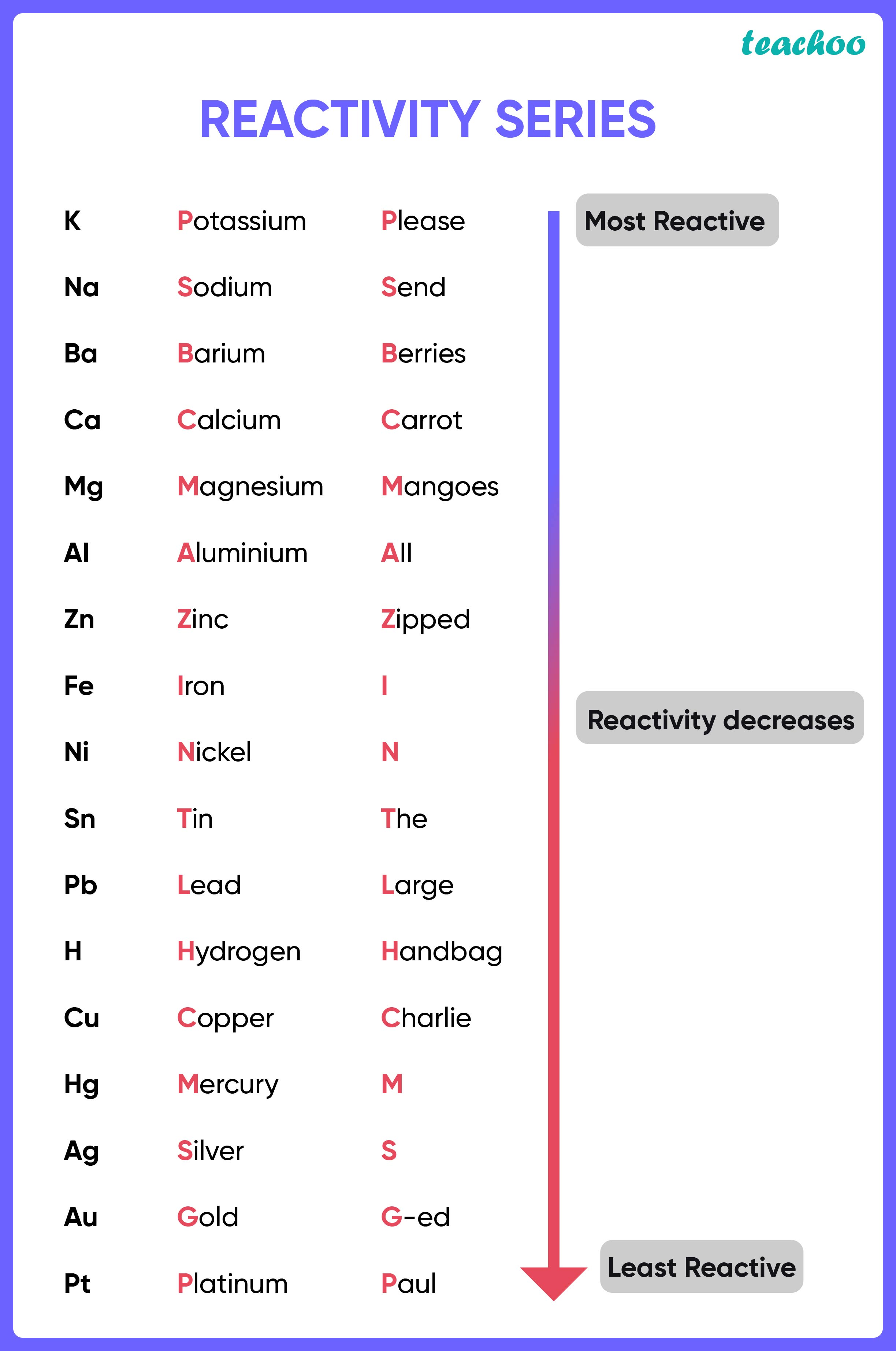Case Based Questions (MCQ)

Class 10
Chapter 1 Class 10 - Chemical Reactions and Equations

## (iv) R and P

• In the first reaction , Q is able to replace P from its salt i.e. P 2 O 3 , Q is more reactive than P.
• In the second reaction, Q is also able to replace R from its salt solution i.e. RSO 4 , Q is more reactive than R .
• In the third reaction, P is able to replace r from its oxide, so, P is more reactive than R

Therefore, the r eactivity order is: Q > P > R ( Q being the most reactive and R being the least)

Thus,

• Most Reactive is Q
• Least Reactive is R

So, the correct answer is  (ii)

## (iv) Substitution reaction

Ans.

Let’s look at all the options

• Displacement Reaction : Reaction in which more reactive element takes the place of less reactive element.
• Combination Reaction : When two or more substances react to form a single product, it is known as combination reaction.
• Neutralisation reaction : A reaction in which acid and base react to form salt and water
• Substitution Reaction: In this reaction, one functional group in a chemical compound is replaced by another.

Since in the above mentioned reactions, the more reactive elements (Q, P) are replacing the less reactive element (R), this is a displacement reaction

So, the correct answer is (i) Displacement reaction

## (iv) none of the above

Ans.

Since in this reaction, Q is able to replace R from its salt RSO 4

Therefore, Q is more reactive than R.

So, the correct answer is (i)

## (iv) Copper and lead are more reactive elements  than zinc.

Ans.

According to the reactivity series,

Zinc and lead are more reactive than copper because they both are able to replace copper from its salt solution.So, the correct answer is (i)

## (iv) None of the above.

Ans.

Since in this reaction the two reacting compounds are switching their positive and negative ions,

it is a double displacement reaction.

So, the correct answer is (i)

Learn in your speed, with individual attention - Teachoo Maths 1-on-1 Class